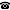# 2014 seminar talk: Folding Up (Disjunctions)

Talk held by Peter Schuster (Pure Mathematics, University of Leeds, UK) at the KGRC seminar on 2014-01-09.

### Abstract

The characteristic axiom of many an ideal object in algebra and logic (prime ideal, ultrafilter, valuation ring, linear order, complete theory, etc.) can be put as a variant of the interpretation of disjunction in a model of classical logic: that is, "if $A\lor B$ holds, then either $A$ holds or $B$ holds". Typically the characteristic axiom can be reduced to the special case that corresponds to disjunction interpretation where $A = B$. Unlike the latter, however, the former is not always a tautology.

The reduction is usually done by an appropriate form of the Axiom of Choice (Krull's Lemma, the Artin-Schreier Theorem, Lindenbaum's Lemma, etc.); whence it falls short of telling us why it works and why $A = B$ matters. In spite of the resulting transfinite flavour, we hope that the reduction can often be done by finite methods only, and that we thus gain reasons and routines on top of the facts. Towards a systematic approach we have singled out a universal Krull-Lindenbaum Theorem crossing algebra and logic.

Partially joint work with F. Ciraulo, N. Gambino and D. Rinaldi.

Kurt Gödel Research Center for Mathematical Logic. Währinger Straße 25, 1090 Wien, Austria.+43-1-4277-50501. Last updated: 2010-12-16, 04:37.